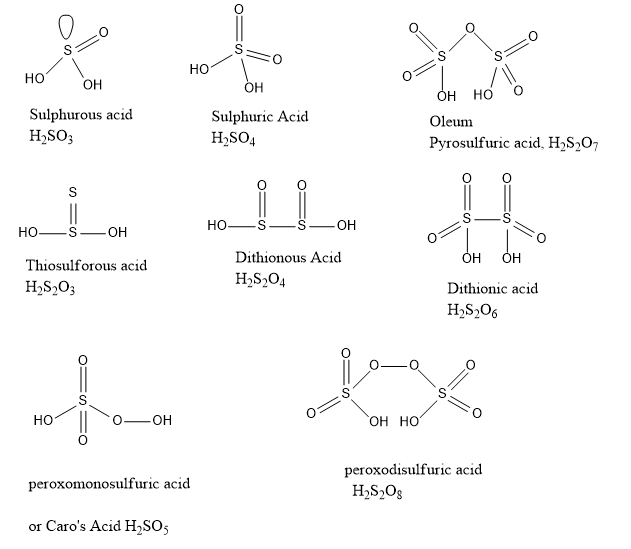Question

Which of the following has O-O linkage :

A
B
C
D
Medium

SolutionVerified by Toppr
Correct option is B)

Correct Option: Hint: Peroxide linkage is generally found in oxoacids with higher oxidation states calculated.Explanation: Consider the highest oxidation state of Sulphur which is . Calculate the oxidation states of Sulphur atoms using the normal calculation method. If the oxidation state is equal to the highest, there is linkage. For example oleum. If the oxidation state calculated is lower than highest, there is linkage for example or . If it is more than the highest possible, there is peroxide linkage, i.e. linkage such as .Hence final answer is Option00